# SAT II Math I : 3-Dimensional Geometry

## Example Questions

← Previous 1 3 4 5 6 7

### Example Question #1 : 3 Dimensional Geometry

A circular swimming pool has diametermeters and depth 2 meters throughout. Which of the following expressions give the amount of water it holds, in cubic meters?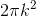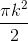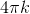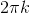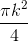Explanation:

The pool can be seen as a cylinder with diameter- and, subsequently, radius half this, or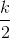- and depth, or height, 2. The volume of a cylinder is defined by the formula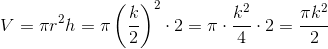### Example Question #1 : Volume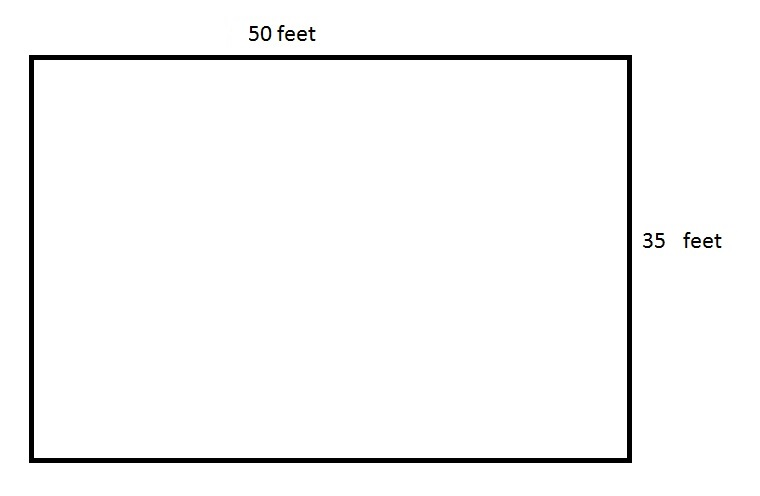The above depicts a rectangular swimming pool for an apartment. 60% of the pool is six feet deep, and the remaining part of the pool is four feet deep. How many cubic feet of water does the pool hold?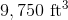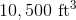None of the other choices gives the correct answer.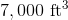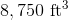None of the other choices gives the correct answer.

Explanation:

The cross-section of the pool is the area of its surface, which is the product of its length and its width: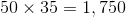square feet.

Since 60% of the pool is six feet deep, this portion of the pool holds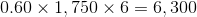cubic feet of water.

Since the remainder of the pool - 40% - is four feet deep, this portion of the pool holds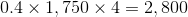cubic feet of water.

Add them together: the pool holds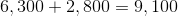cubic feet of water.

This answer is not among the choices.

### Example Question #1 : Volume

Find the volume of a cube in inches with a side of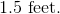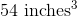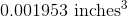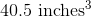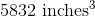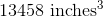Explanation:

Convert the side dimension to inches first before finding the volume.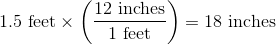Write the volume for a cube and substitute the new side to obtain the volume in inches.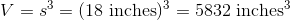### Example Question #4 : Volume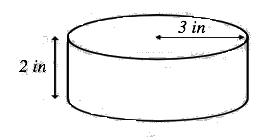Figure not drawn to scale.

What is the volume of the cylinder above?

66.13 in3

48.79 in3

52.36 in3

56.55 in3

94.25 in3

56.55 in3

Explanation:

In order to find the volume of a cylinder, you find the area of the circular top and multiply it by the height.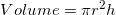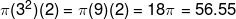### Example Question #5 : Volume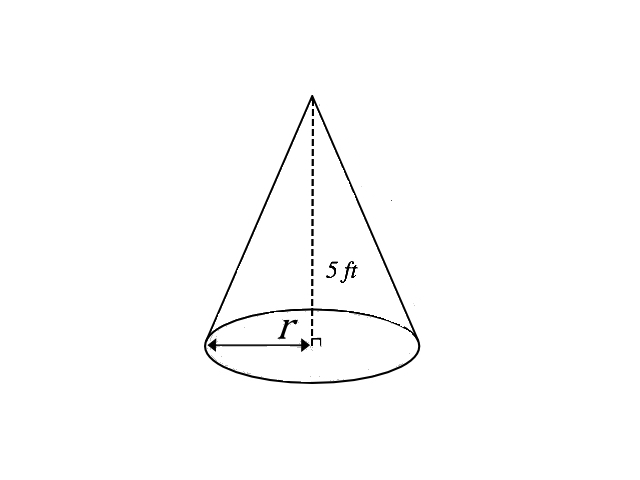Figure not drawn to scale

# If the volume of the cone above is 47.12 ft3, what is the radius of the base?

3.5ft

3 ft

2 ft

4 ft

5 ft

3 ft

Explanation:

Because we have been given the volume of the cone and have been asked to find the radius of the base of the cone, we must work backwards using the volume formula.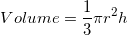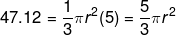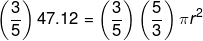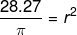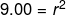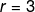### Example Question #6 : Volume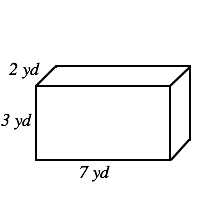Figure not drawn to scale.

What is the volume of the above image?

42 yd2

32 yd3

64 yd3

84 yd2

42 yd3

42 yd3

Explanation:You can find the volume of a box by following the equation below: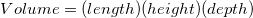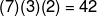### Example Question #1 : How To Find The Volume Of A Tetrahedron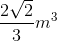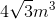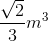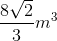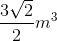Explanation:

A regular tetrahedron is composed of four equilateral triangles. The formula for the volume of a regular tetrahedron is: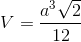, whererepresents the length of the side.

Plugging in our values we get: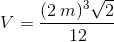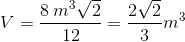### Example Question #2 : How To Find The Volume Of A Tetrahedron

Find the volume of a tetrahedron with an edge of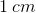.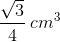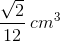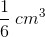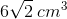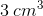Explanation:

Write the formula for the volume of a tetrahedron.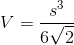Substitute in the length of the edge provided in the problem.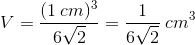Rationalize the denominator.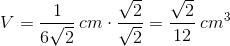### Example Question #3 : How To Find The Volume Of A Tetrahedron

Find the volume of a tetrahedron with an edge of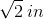.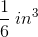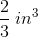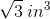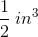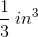Explanation:

Write the formula for the volume of a tetrahedron.Substitute in the length of the edge provided in the problem: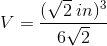Cancel out thein the denominator with one in the numerator: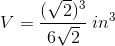A square root is being raised to the power of two in the numerator; these two operations cancel each other out. After canceling those operations, reduce the remaining fraction to arrive at the correct answer: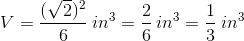### Example Question #4 : How To Find The Volume Of A Tetrahedron

Find the volume of a tetrahedron with an edge of.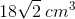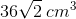Explanation:

Write the formula for finding the volume of a tetrahedron.Substitute in the edge length provided in the problem.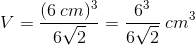Cancel out thein the denominator with part of the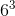in the numerator: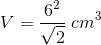Expand, rationalize the denominator, and reduce to arrive at the correct answer: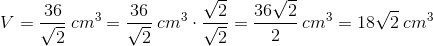← Previous 1 3 4 5 6 7